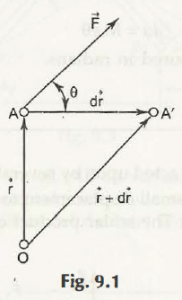Consider a particle moves from one point A to another point A’ due to an external force F as shown in Fig. 9.1Here,

Vector AA’ = dr = Displacement of particle

Position vector of A is Vector r

Position vector of A’ is Vector r + dr

Then,

Work, du can be given by-

du = F . dr

i.e.,

Magnitude of work is the product of force and displacement.

|F| = F and |dr| = ds

For dot product, it can be written as-

du = F ds cos

where,

= Angle between F and d

It defines the magnitude of work is the product of component of force and displacement. The component of force should be in the direction of displacement.

Links of Next Mechanical Engineering Topics:-### Customer Reviews

My Homework Help
Rated 5.0 out of 5 based on 510 customer reviews at